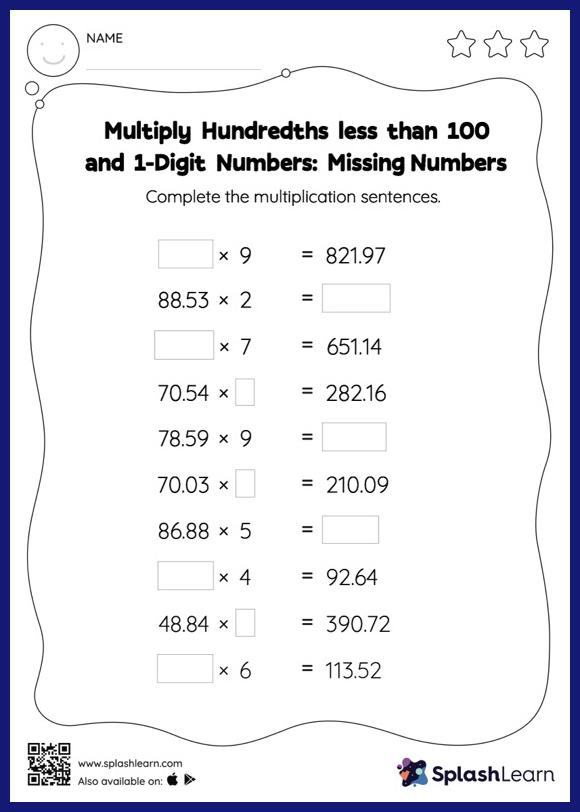# Multiply Hundredths less than 100 and 1-Digit Numbers: Missing Numbers Worksheet

Home > Multiply Hundredths less than 100 and 1-Digit Numbers: Missing NumbersThis multiply hundredths less than 100 and 1-digit numbers worksheet consists of a set of questions on multiplication, which help students develop fluency. Students may omit the decimal point when multiplying a decimal by a one-digit number and then add it when writing the answer. Students find missing numbers in multiply hundredths less than 100 and 1-digit numbers worksheet using this concept. In each problem, the numbers are laid out in the horizontal format. Students should try to use different strategies involving composing and decomposing numbers to solve these problems. This will help them develop flexibility and fluency.